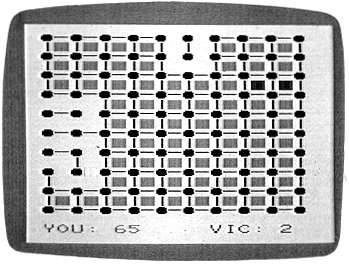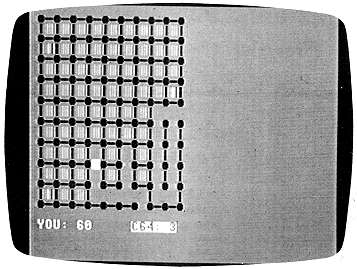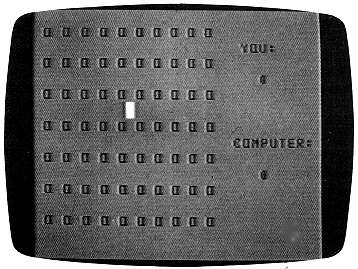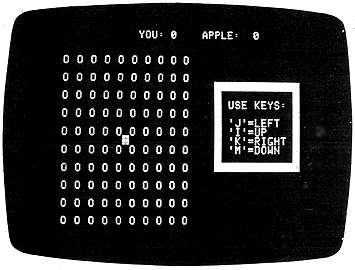`COMPUTE! ISSUE 40 / SEPTEMBER 1983 / PAGE 128`

DOTS

Eric K. Evans

Easy to play, but challenging, this game pits you against your computer. You can choose one of ten skill levels. Written for the VIC with versions for the 64, Color Computer, and Apple.

"Dots" is based on a strategy game that many people first come across while they are in elementary or junior high school. You remember it: you and a friend take a couple of pencils and a piece of paper and draw several rows of dots on the paper. Then you take turns drawing horizontal or vertical lines that would connect two dots. These lines form the sides of squares.
The object of the game is to maneuver your friend into drawing the third side of a square so that you can draw the fourth side and complete the square. Every time you finish a square you get one point and another turn. When all of the squares are finished, the game ends and the person with the most points wins.
With this program, your computer will be the friend you play against and it will also act as umpire. Its decisions will be final, but don't worry - it doesn't cheat.

How To Play
The first thing you need to do after typing in or loading the game is enter either a J if you plan to use a joystick for input or a K if you are going to use the keyboard cursor control keys and the RETURN key for input. Next you will be asked to enter a skill level between 0 and 10 (with 10 being the most difficult).
If you enter 0 as your skill level, the VIC will play randomly and you should win with little effort. At skill level 10, be prepared for a real strategic challenge. At level 0, the VIC will make its move immediately after yours. As the level of play increases, the VIC's response time increases, too. At level 10, it will usually take between 5 and 20 seconds to make its move.
When the game starts, a 100-dot (ten by ten) game board will be displayed with a scoring area at the bottom of the screen. You always move first. To move, position the yellow cursor where you want to draw a line, and then hit the joystick fire button or the RETURN key to enter your move. When the VIC makes a move, it will beep and flash the line it is going to draw to make sure you see where it is moving.
Whenever you complete a square, the square is colored in cyan and you get another turn. Whenever the VIC completes a square, the square is colored in red and the VIC moves again. These colors are used because they contrast well on black-and-white as well as color TVs. The game continues until all 81 squares have been formed and the winner is declared.
If you don't want to spend the time to type in this program, send me a cassette, a self-addressed, stamped mailer, and \$3, and I will return your tape with two copies (just to be safe) of the game on it. This is only for the VIC version.

Eric K. Evans
P.O. Box 6287
New Haven, CT 06520

 Color Computer And Apple Version Notes For Dots The object of Dots on the Color Computer and the Apple is to form more squares than the computer by connecting dots with horizontal and vertical lines. On the Color Computer, the game is played on a 70-dot grid. Move the yellow cursor around the game board with the arrow keys. When it is in a position where you wish to draw a line, press the ENTER key. Squares that you complete will be colored orange; those the computer captures are colored red.     Dots on the Apple features a 100-dot game board and is played like the Color Computer version. A flashing asterisk, moved with the J,I,K, and M keys, indicates your position. Press the RETURN key to draw a connecting line. When a square is formed, an inverse Y or A is displayed, crediting either you or the Apple with the capture.An almost completed game of "Dots," VIC version.

Program 1: Dots - VIC Version

10 PRINT"{CLR}{2 DOWN}"SPC(18)"DOTS
{DOWN}"
20 INPUT"{6 SPACES}JOYSTICK{DOWN)
{5 LEFT}OR{DOWN}{8 LEFT}KEYBOARD(J/K)
";A\$
30 JK=-1:IFA\$="K"THENJK=0
40 INPUT"{3 DOWN}{RIGHT}SKILL LEVEL(0-10
)";SK:IFSK<0ORSK>10THEN40
50 SK=(10-SK)/10:TS=200-200*SK:DT=TS+25:
DD=37154:POKE37139,0:POKE36879,29
60 SC=4*(PEEK(36866)AND128)+64*(PEEK(368
69)AND120):C0=37888+4*(PEEK(36866)AND
128)
70 PRINT"{CLR}":FORI=1TO10:PRINT"{BLK} Q
Q Q Q Q Q Q Q Q Q{DOWN}":NEXT
80 YS=0:VS=0:PRINT" {CYN}YOU:"YS"
{4 SPACES}{RED}VIC:"VS
90 DEFFNBX(LC)=(PEEK(LC+22)<>32)+(PEEK(L
C+1)<>32)+(PEEK(LC-22)<>32)+(PEEK(LC-
1)<>32)
100 DEFFNVH(LC)=LC<>2*INT(LC/2)
110 SL=SC+230:CL=CO+230:X=10:Y=10:CC=PEE
K(SL):CR=PEEK(CL)
120 POKESL,160:POKECL,7:F=0
130 IFJKTHEN150
140 GOSUB930:GOTO160
150 GOSUB860:IFPC=0THEN150
160 X=X+J:Y=Y+K:IFX<1ORX>190RY<1ORY>19TH
ENX=X-J:Y=Y-K:GOTO130
170 IFPC=99THEN200
180 POKESL,CC:POKECL,CR:SL=SL+PC:CL=CL+P
C:CC=PEEK(SL):POKESL,160
190 CR=PEEK(CL):POKECL,7:GOTO130
200 L=(PEEK(SL+1)=81)+(PEEK(SL-1)=81)+(P
EEK(SL+22)=81)+(PEEK(SL-22)=81)
210 IFL+(CC=32)=-3THEN230
220 I=128:GOSUB710:GOTO130
230 WH=1:ML=SL:GOSUB650:IFNOTFNVH(SL)THE
N280
240 IFX>lANDFNBX(SL-1)=-4THENBX=SL-1:GOS
UB720:F=-1
250 IFX<19ANDFNBX(SL+1)=-4THENBX=SL+1:GO
SUB720:GOTO110
260 IFFTHEN110
270 GOTO310
280 IFY>lANDFNBX(SL-22)=-4THENBX=SL-22:G
OSUB720:F=-1
290 IFY<19ANDFNBX(SL+22)=-4THENBX=SL+22:
GOSUB720:GOTO110
300 IFFTHEN110
310 WH=2:F=0:CN=0:IFRND(0)<SKTHEN390
320 FORI=44TO396STEP44:FORJ=2TO18STEP2:K
=SC+I+J
330 IFPEEK(K)=32ANDFNBX(K)=-3THEN350
340 NEXTJ,I:GOTO390
350 I=K:IFPEEK(I-22)=32THENI=I-22:GOTO54
0
360 IFPEEK(I+22)=32THENI=I+22:GOTO540
370 IFPEEK(I-1)<>32THENI=I+1:GOTO600
380 IFPEEK(I+1)<>32THENI=I-1:GOTO600
390 I=INT(RND(O)*415+SC+23):CN=CN+1:7FPE
EK(I)<>32THEN390
400 IFNOT((PEEK(I+1)=81ANDPEEK(I-1)=81)0
R(PEEK(I+22)=81ANDPEEK(I-22)=81'))THE
N390
410 IFSK>.60RCN>TSTHEN470
420 IFFNVH(I)THEN450
430 IFFNBX(I-22)=-20RFNBX(I+22)=-2THEN39
0
440 GOTO540
450 IFFNBX(I-1)=-20RFNBX(I+1)=-2THEN390
460 GOTO600
470 IFFNVH(I)THEN510
480 IFSK>.60RCN>DTTHEN540
490 IFFNBX(I+22)=-2ANDFNBX(I-22)=-2THEN3
90
500 GOTO540
510 IFSK>.6ORCN>DTTHEN600
520 IFFNBX(I+1)=-2ANDFNBX(I-1)=-2THEN390
530 GOTO600
540 ML=I:GOSUB650
550 IFFNBX(ML-22)=-4THENBX=ML-22:GOSUB72
0:F=-1
560 IFFNBX(ML+2?2)=-4THENBX=ML+22:GOSUB72
0:GOTO310
570 IFFTHEN310
580 GOTO110
590 IFNOT(PEEK(I-22)=81ANDPEEK(I+22)=81)
THEN390
600 ML=I:GOSUB650
610 IFFNBX(ML-1)=-4THENBX=ML-1:GOSUB720:
F=-1
620 IFFNBX(ML+1)=-4THENBX=ML+1:GOSUB720:
GOTO310
630 IFFTHEN310
640 GOTO110
650 CL=CO+ML-SC
660 POKEML,67
670 IFFNVH(ML)THENPOKEML,93
680 I=185:IFWH=2THENI=150
690 FORJ=ITOWH:POKECL,0:GOSUB710:POKECL,
1
700 FORL=1TO200:NEXT:POKECL,0:NEXT
710 POKE36878,15:POKE36876,I:FORK=1TO200
:NEXT:POKE36878,0:POKE36876,0:RETURN
720 YS=YS+1:J=3:I=200:CL=CO+BX-SC:IFWH=2
THENJ=2:I=150:YS=YS-1:VS=VS+1
730 POKEBX,160:POKECL,1
740 FORL=1TO3:POKECL,J:GOSUB710:POKECL,1
:FORK=1TO200:NEXT:I=I+18:POKECL,J:NE
XT
750 PRINT"{HOME}{21 DOWN) {CYN}YOU:"YS"
{4 SPACES}{RED}VIC:"VS
760 IFYS+VS<81THENRETURN
770 PRINT"{HOME}{19 DOWN}":IFYS>VSTHEN80
0
780 PRINT"{BLK}{7 SPACES}YOU LOSE"
790 POKE36878,15:POKE36877,128:FORI=1TO1
500:NEXT:GOTO850
800 PRINT"{DOWN}":FORJ=1TO3
810 PRINT"{2 UP}{RVS}{BLU}I{RED}**{BLU}I
{PUR}{3 SPACES}YOU{2 SPACES}WIN
{3 SPACES}{BLU}I{RED}**{BLU}I"
820 POKE36878,15:FORL=128TO255:POKE36876
,L:NEXT:POKE36874,0:POKE36878,0
830 PRINT"{2 UP}{22 SPACES}":FORL=1TO300
:NEXT
840 PRINT"{2 UP}{RVS}{BLU}!{RED}**{BLU}!
{PUR}{3 SPACES}YOU{2 SPACES}WIN
{3 SPACES}{BLU}!{RED}**{BLII}!":NEXT
850 POKE36878,0:POKE36877,0:END
860 J=0:K=0:PC=0:POKEDD,127:IFPEEK(37152
)=119THENPC=1:J=1:POKEDD,255:RETURN
870 POKEDD,255:I=PEEK(37137)
880 IF(IAND4)=0THENPC=-22:K=-1:RETURN
890 IF(IAND8)=0THENPC=22:K=1:RETURN
900 IF(IAND16)=0THENPC=-l:J=-1:RETURN
910 IF(IAND32)=0THENPC=99
920 RETURN
930 J=0:K=0:PC=0
940 GETA\$:IFA\$=""THEN940
950 IFA\$="{UP}"THENPC=-22:K=-l:RETURN
960 IFA\$="{RIGHT}"THENJ=1:PC=1:RETURN
970 IFA\$="{DOWN}"THENK=1:PC=22:RETURN
980 IFA\$="{LEFT}"THENJ=-l:PC=-1:RETURN
990 IFASC(A\$)=13THENPC=99
1000 RETURN"DOTS," 64 version. The computer has almost lost the game.

Program 2: Dots - 64 Version

5 POKE 53281,12:POKE53280,0
10 PRINT"{BLK}{RVS}{CLR}{2 DOWN}"SPC(17)
"DOTS
20 PRINT"(2 DOWN}{15 SPACES}{WHT)JOYSTIC
K{DOWN}{5 LEFT}OR{DOWN}{7 LEFT}KEYBOA
RD(J/K){SHIFT-SPACE}?";
25 GETA\$:IFA\$<>"J"ANDA\$<>"K"THEN25
26 PRINTA\$
30 JK=-l:IFA\$="K"THENJK=0
40 INPUT"{3 DOWN}{RIGHT}SKILL LEVEL(0-10
)";SK:IFSK<0ORSK>10THEN40
50 SK=(10-SK)/10:TS=200-200*SK:DT=TS+25
60 SC=1024 : CO = 13*4096+8*256,
70 PRINT"{CLR}":FORI=1TO10:PRINT"IBLK} Q
Q Q Q Q Q Q Q Q Q{DOWN}":NEXT
80 YS=0:VS=0:PRINT" {WHT}YOU:"YS"
{4 SPACES}{RVS}C64:"VS
90 DEFFNBX(LC)=(PEEK(LC+40)<>32)+(PEEK(L
C+1)<>32)+(PEEK(LC-40)<>32)+(PEEK(LC-
1)<>32)
100 DEFFNVH(LC)=LC<>2*INT(LC/2)
110 SL=SC+450:CL=CO+450:X=10:Y=11:CC=PEE
K(SL):CR=PEEK(CL)
120 POKESL,160:POKECL,7:F=0
130 IFJKTHEN150
140 GOSUB930:GOTO160
150 GOSUB860:IFPC=0THEN150
160 X=X+J:Y=Y+K:IFX<IORX>190RY<1ORY>19TH
ENX=X-J:Y=Y-K:GOTO130
170 IFPC=99THEN200
180 POKESL,CC:POKECL,CR:SL=SL+PC:CL=CL+P
C:CC=PEEK(SL):POKESL,160
190 CR=PEEK(CL):POKECL,7:GOTO130
200 L=(PEEK(SL+1)=81)+(PEEK(SL-1)=81)+(P
EEK(SL+40)=81)+(PEEK(SL-40)=81)
210 IFL+(CC=32)=-3THEN230
220 I=128:GOSUB710:GOTO130
230 WH=1:ML=SL:GOSUB650:IFNOTFNVH(SL)THE
N280
240 IFX>lANDFNBX(SL-1)=-4THENBX=SL-1:GOS
UB720:F=-1
250 IFX<19ANDFNBX(SL+1)=-4THENBX=SL+1:GO
SUB720:GOTO110
260 IFFTHEN110
270 GOTO310
280 IFY>lANDFNBX(SL-40)=-4THENBX=SL-40:G
OSUB720:F=-I
290 IFY<19ANDFNBX(SL+40)=-4THENBX=SL+40:
GOSUB720:GOTO110
300 IFFTHEN110
310 WH=2:F=0:CN=0:IFRND(0)<SKTHEN390
320 FORI=80TO720STEP80:FORJ=2TO18STEP2:K
=SC+I+J
330 IFPEEK(K)=32ANDFNBX(K)=-3THEN350
340 NEXTJ,I:GOTO390
350 I=K:IFPEEK(I-40)=32THENI=I-40:GOTO54
0
360 IFPEEK(I+40)=32THENI=I+40:GOTO540
370 IFPEEK(I-1)<>32THENI=I+1:GOTO600
380 IFPEEK(I+1)<>32THENI=I-1:GOTO600
390 I=INT(INT(RND(0)*20)*40+21*RND(O)+SC
+41):CN=CN+1:IFPEEK(I)<>32THEN390
400 IFNOT((PEEK(I+1)=81ANDPEEK(I-1)=81)0
R(PEEK(I+40)=81ANDPEEK(I-40)=81))THE
N390
410 IFSK>.6ORCN>TSTHEN470
420 IFFNVH(I)THEN450
430 IFFNBX(I-40)=-20RFNBX(I+40)=-2THEN39
0
440 GOTO540
450 IFFNBX(I-1)=-20RFNBX(I+1)=-2THEN390
460 GOTO600
470 IFFNVH(I)THEN510
480 IFSK>.6ORCN>DTTHEN540
490 IFFNBX(I+40)=-2ANDFNBX(I-40)=-2THEN3
90
500 GOTO540
510 IFSK>.6ORCN>DTTHEN600
520 IFFNBX(I+1)=-2ANDFNBX(I-I)=-2THEN390
530 GOTO600
540 ML=I:GOSUB650
550 IFFNBX(ML-40)=-4THENBX=ML-40:GOSUB72
0:F=-1
560 IFFNBX(ML+40)=-4THENBX=ML+40:GOSUB72
0:GOTO310
570 IFFTHEN310
580 GOTO110
590 IFNOT(PEEK(I-40)=81ANDPEEK(I+40)=81)
THEN390
600 ML=I:GOSUB650
610 IFFNBX(ML-1)=-4THENBX=ML-1:GOSUB720:
F=-1
620 IFFNBX(ML+1)=-4THENBX=ML+1:GOSUB720:
GOTO310
630 IFFTHEN310
640 GOTO110
650 CL=CO+ML-SC
660 POKEML,67
670 IFFNVH(ML)THENPOKEML,93
680 I=185:IFWH=2THENI=150
690 FORJ=ITOWH:POKECL,O:GOSUB710:POKECL,
1
700 FORL=1TO200:NEXT:POKECL,0:NEXT
710 FORK=1T0200:NEXT:RETURN
720 YS=YS+1:J=3:I=200:CL=CO+BX-SC:IFWH=2
THENJ=2:I=150:YS=YS-1:VS=VS+1
730 POKEBX,160:POKECL,1
740 FORL=1TO3:POKECL,J:GOSUB710:POKECL,1
:FORK=170200:NEXT:I=I+18:POKECL,J:NE
XT
750 PRINT"{HOME}{21 DOWN} {WHT}YOU:"YS"
{4 SPACES}{RVS}C64:"VS
760 IFYS+VS<81THENRETURN
770 PRINT"{HOME}{19 DOWN}":IFYS>VSTHEN80
0
780 PRINT"{BLK}{7 SPACES}YOU LOSE
{7 SPACES)"
790 GOTO850
800 PRINT"{DOWN}YOU WIN !!!"
850 END
860 J=0:K=0:PC=0
870 I=PEEK(56321)
880 IF(IAND1)=0THENPC=-40:K=-1:RETURN
885 IF(IANDS)=0THENJ=1:PC=1:RETURN
890 IF(IAND2)=0THENPC=40:K=1:RETURN
900 IF(IAND4)=0THENPC=-1:J=-1:RETURN
910 IF(IAND16)=0THENPC=99
920 RETURN
930 J=0:K=0:PC=0
940 GETA\$:IFA\$=""THEN940
950 IFA\$="{UP}"THENPC=-40:K=-1:RETURN
960 IFA\$="{RIGHT}"THENJ=I:PC=1:RETURN
970 IFA\$="{DOWN}"THENK=1:PC=40:RETURN
980 IFA\$="{LEFT}"THENJ=-1:PC=-1:RETURN
990 IFASC(A\$)=13THENPC=99
1000 RETURNNo moves have been made in this Color Computer version
of "DOTS."

Program 3: Dots - Color Computer Version
Translation by Patrick Parrish, Editorial Programmer

100 CLS 7:PRINT@236, "{REV}D{OFF} {REV}O{OFF} {REV}T{OFF} {REV}S{OFF}";
110 PRINT@357,"SKILL LEVEL (0-10)";
:INPUT SK: IF SK<0 OR SK>10 THEN
110
120 CLS:SK=(10-SK)110:TS=200-200*Sk;
:DT=TS+25:SC=1024
130 PRINT:FOR I=1 TO 7:PRINT" O O O
O O O O O O O":PRINT:NEXT I
140 YS=0:CS=0:PRINT@87,"YOU:":PRINT
@278,"COMPUTER:"
150 PRINT@152,YS:PRINT@344,CS
160 DEF FNBX(LC)=(PEEK(LC+32)<>96)+
(PEEK(LC+1)<>96)+(PEEK(LC-32)<>
96)+(PEEK(LC-1)<>96)
170 DEF FNVH(LC)=(LC<>2*INT(LC/2))
180 SL=SC+202:X=10:Y=7:CC=PEEK(SL)
190 POKE SL,128+16*(2-1)+15:F=0
200 GOSUB 910
210 X=X+J:Y=Y+K:IFX<1ORX>19ORY<2ORY
>14THEN X=X-J:Y=Y-K::GOTO 200
220 IF PC=99 THEN 250
230 POKE SL,CC:SL=SL+PC:CC=PEEK(SL)
:POKE SL,128+16*(2-1)+15
240 GOTO 200
250 L=(PEEK(SL+1)=79)+(PEEK(SL-1)=7
9)+(PEEK(SL+32)=79)+(PEEK(SL-32
)=79)
260 IF L+(CC=96)=-3 THEN 280
270 I=62:GOSUB 760:GOTO 200
280 WH=1:ML=SL:GOSUB 700:IF NOT FNV
H(SL)THEN 330
290 IF X:>1 AND FNBX(SL-1)=-4 THEN H
X=SL-1:GOSUB 770:F=-1
300 IF X<19 AND FNBX(SL+1)=-4 THEN
BX=SL+I:GOSUB 770:GOTO 180
310 IF F THEN 180
320 GOTO 360
330 IF Y:r1 AND FNBX(SL-32)=-4 THEN
BX=SL-32:GOSUB 770:F=-1
340 IF Y<13 AND FNBX(SL+32)=-4 THEN
BX=SL+-2:GOSUB 770:GOTO 180
350 IF F THEN 180
360 WH=2:F=0:CN=0:IF RND(0)<SK THEN
440
370 FOR I=64 TO 384 STEP 64:FOR J=2
TO 12 STEP 2:k:=SC+I+J
380 IFPEEK(K)=96ANDFNBX(K)=-3 THEN
400
390 NEXTJ,I:GOTO 440
400 I=K:IF PEEK(I-32)=96 THEN I=I-3
2:GOTO 590
410 IF PEEK(I+32)=96 THEN I=I+32:60
TO 590
420 IF PEEK(I-1)<>96 THEN I=I+I:GOT
O 650
430 IF PEEK(I+1)<>96 THEN I=I-1:GOT
O 650
440 I=RND(19)+RND(13)*32+33+SC:CN=C
N+1:IF PEEK(I)<>96THEN 440
450 IFNOT((PEEK(I+1)=79ANDPEEK(I-1)
=79)OR(PEEK(I+32)=79ANDPEEK(I-3
2)=79))THEN 440
460 IF SK>.6 OR CN>TS THEN 520
470 IF FNVH(I) THEN 500
480 IF FNBX(I-32)=-2 OR FNBX(I+=2)=
-2 THEN 440
490 GOTO 590
500 IF FNBX(I-1)=-2 OR FNBX(I+-1)=-2
THEN 440
510 GOTO 650
520 IF FNVH(I) THEN 560
530 IF SK>.6 OR CN>DT THEN 590
540 IF FNBX(I+-2)=-2 AND FNBX(I-32)
=-2 THEN 440
550 GOTO 590
560 IF SK>.6 OR CN>DT THEN 650
570 IF FNBX(I+1)=-2 AND FNBX(I-1)=-
2 THEN 440
580 GOTO 650
590 ML=I:GOSUB 700
600 IF FNBX(ML-32)=-4 THEN BX=ML-32
:GOSUB 770:F=-1
610 IF FNBX(ML+32)=-4 THEN BX=ML+32
GOSUB 770:GOTO -60
620 IF F THEN 360
630 GOTO 180
640 IF NOT (PEEK:(I-32)=79 AND PEEK(
I+32)=79) THEN 440
650 ML=I:GOSUB 700
660 IF FNBX(ML-1)=-4 THEN BX=ML-1:G
OSUB 770:F=-1
670 IF FNBX(ML+1)=-4 THEN BX=ML+1:G
OSUB 770:GOTO 360
680 IF F THEN 360
690 GOTO 180
700 REM
710 POKE ML,109
720 IF FNVH(ML) THEN POKE ML,73
730 I=185:IF WH=2 THEN I=150.
740 FOR J=1 TO WH:GOSUB 760
750 FOR L=1 TO 200:NEXT:NEXT
760 SOUND I,2:RETURN:REM NOISE
770 YS=YS+1:J=8:I=200:IF WH=2 THEN
J=4:I=150:YS=YS-1:CS=CS+1
780 POKE BX,128+16*(5-1)+15
790 FOR L=1 TO 3:POKE BX,128+16*(J-
1)+15:GOSUB 760:POKE.BX,128+16*
(5-1)+15:FOR I=1 TO 200:NEXT:I=
I+18:POKE BX,128+16*(J-1)+15:NE
XT
800 PRINT@152,YS:PRINT@344,CS
810 IF YS+CS<54 THEN RETURN
820 IF YS>CS THEN 850
830 PRINT@487,"SORRY, YOU LOST.";
840 SOUND 128,10:FOR1=1 TO 1500:NEX
T I:GOTO 870
850 PRINT@490,"YOU WIN!!!!!";
660 FOR I=128 TO 255:SOUND I,1:NEXT
870 PRINT@487,"PLAY AGAIN ({REV}Y/N{OFF}) ?";
880 A\$=INKEY\$:IF A\$="" THEN 880
890 IF LEFT\$(A\$,1)="Y" THEN 100
900 END
910 J=0:K=0:PC=0
920 A\$=INKEY\$:IF A\$="" THEN 920
930 IF A\$=CHR\$(94) THEN PC=-32:K=-1
:RETURN
940 IF A\$=CHR\$(9) THEN J=1:PC=I:RET
URN
950 IF A\$=CHR\$(10) THEN K=1:PC=32:R
ETURN
960 IF A\$=CHR\$(8) THEN J=-1:PC=-1:R
ETURN
970 IF ASC(A\$)=13 THEN PC=99
980 RETURNThis game is just beginning in the Apple version of "Dots."

Program 4: Dots -Apple Version
Translation by Patrick Parrish, Editorial Programmer

100 DIM XL%(23): FOR I = 0 TO 7:XL%(I)
= 1024 + 128 * I:XL%(I + 6) = 106
4 + 128 * I:XL%(I + 16) = 1104 + 1
28 * I: NEXT I
110 FOR I = 770 TO 795: READ M: POKE I
,M: NEXT
120 TEXT : HOME : VTAB 11: HTAB 19: INVERSE
:PRINT "D O T S": FOR J = 1 TO 10
00: NEXT J: NORMAL
130 VTAB 15: HTAB 11: INPUT "SKILL LEV
EL (0-10) ?";SK: IF SK < 0 OR SK >
10 THEN 130
140 SK = (10 - SK) / 10:TS = 200 - 200 *
SK: DT = TS + 25
150 HOME : PRINT : PRINT : FOR I = 1 TO
10: PRINT : PRINT " O O O O O O O
O O O": NEXT
160 VTAB 9: HTAB 27: PRINT "USE KEYS:"
: VTAB 11: HTAB 27: PRINT "'J'=LEF
T": HTAB 27: PRINT "'I'=UP": HTAB
27: PRINT "'K'=RIGHT": HTAB 27: PRINT
"'M'=DOWN"
170 VTAB 7: HTAB 25: INVERSE : PRINT "
": VTAB 16: HTAB 25: PRINT
"             "
180 FOR ROW = 7 TO 14: FOR COL = 24 TO
36 STEP 12: POKE XL%(ROW) + COL,32
: NEXT : NEXT : NORMAL
190 YS = 0:AS = 0: VTAB 1: HTAB 13: PRINT
"YOU: "YS"    APPLE: "AS;
200 DEF FN BX(COL) = - ( PEEK (XL%(R
OW + 1) + COL) < > 160) - ( PEEK
(XL%(ROW) + COL + 1) < > 160) -
PEEK (XL%(ROW - 1) + COL) < > 16
0) - ( PEEK (XL%(ROW) + COL - 1) <
> 160)
210 DEF  FN BY(ROW) = - ( PEEK (XL%(R
OW + 1) + COL) <  > 160) - ( PEEK
(XL%(ROW) + COL + 1) <  > 160) - (
PEEK (XL%(ROW - 1) + COL) <  > 16
0) - ( PEEK (XL%(ROW) + COL - 1) <
> 160)
220 DEF FN VH(ROW) = - ((XL%(ROW) +
COL) < > 2 * INT ((XL%(ROW) + CO
L) / 2))
230 X = 10:Y = 10:ROW = 12:COL = 10:CC =
PEEK (XL% (ROW) + COL)
240 POKE XL% (ROW) + COL, 106: F = 0
250 OLDROW = ROW:OCOL = COL: GOSUB 900
260 X = X + J:Y = Y + K: IF X < 1 OR X >
19 OR Y < 1 OR Y > 19 THEN X = X -
J:Y = Y - K: GOTO 250
270 IF PC = 99 THEN 300
280 POKE XL%(OLDROW) + OCOL,CC:CC = PEEK
(XL%(ROW) + COL): POKE XL%(ROW) +
COL,42
290 GOTO 250
300 L = - ( PEEK (XL%(ROW) + COL + 1) =
207) - ( PEEK (XL%(ROW) + COL - 1)
= 207) - ( PEEK (XL%(ROW + 1) + C
OL) = 207) - ( PEEK (XL%(ROW - 1) +
COL) = 207)
310 IF L - (CC = 160) = - 3 THEN 330
320 POKE 768,250: POKE 769,1: CALL 770
: GOTO 250
330 WH = 1:MROW = ROW:MCOL = COL: GOSUB
750: IF NOT FN VH(ROW) THEN 380
340 BY = ROW: IF X > 1 AND FN BX(COL -
1) = - 4 THEN BX = COL - 1: GOSUB
790:F = - 1
350 IF X < 19 AND FN BX(COL + 1) = -
4 THEN BX = COL + 1: GOSUB 790: GOTO
230
360 IF F = - 1 THEN 230
370 GOTO 410
380 BX = COL: IF Y > 1 AND FN BY(ROW -
1) = - 4 THEN BY = ROW - 1: GOSUB
790:F = - 1
390 IF Y < 19 AND FN BY(ROW + 1) = -
4 THEN BY = ROW + 1: GOSUB 790: GOTO
230
400 IF F = - 1 THEN 230
410 WH = 2:F = 0:CN = 0: IF RND (1) <
SK THEN 490
420 FOR ROW = 4 TO 22 STEP 2: FOR COL =
2 TO 18 STEP 2:K = XL%(ROW) + COL
430 IF PEEK (K) = 160 AND FN BX(COL)
= - 3 THEN 450
440 NEXT : NEXT : GOTO 490
450 I = K: IF PEEK (XL%(ROW - 1) + COL
= 160 THEN ROW = ROW - 1: GOTO 6
40
460 IF PEEK (XL%(ROW + 1) + COL) = 16
0 THEN ROW = ROW + 1: GOTO 640
470 IF PEEK (XL%(ROW) + COL - 1) < >
160 THEN COL = COL + 1: GOTO 700
480 IF PEEK (XL%(ROW) + COL + 1) < >
160 THEN COL = COL - 1: GOTO 700
490 ROW = INT ( RND (1) * 19) + 3:COL =
INT ( RND (1) * 19) + 1:CN = CN +
1 : IF PEEK    (XL%(ROW) + COL) < >
160 THEN 490
500 IF NOT (( PEEK (XL%(ROW) + COL +
1) = 207 AND PEEK (XL%(ROW) + COL
- 1) = 207) OR ( PEEK (XL%(ROW +
1) + COL) = 207 AND PEEK (XL%(ROW
- 1) + COL) = 207)) THEN 490
510 IF SK > .6 OR CN > TS THEN 570
520 IF FN VH(ROW) = - 1 THEN 550
530 IF FN BY(ROW - 1) = - 2 OR FN B
Y(ROW + 1) = - 2 THEN 490
540 GOTO 640
550 IF FN BX(COL - 1) = - 2 OR FN B
X(COL + 1) = - 2 THEN 490
560 GOTO 700
570 IF FN VH(ROW) = - 1 THEN 610
580 IF SK > .6 OR CN > DT THEN 640
590 IF FN BY(ROW + 1) = - 2 AND FN
BY(ROW - 1) = - 2 THEN 490
600 GOTO 640
610 IF SK > .6 OR CN > DT THEN 700
620 IF FN BX(COL + 1) = - 2 AND FN
BX(COL - 1) = - 2 THEN 490
630 GOTO 700
640 MROW = ROW:MCOL = COL: GOSUB 750
650 BX = COL: IF FN BY(ROW - 1) = - 4
THEN BY = ROW - 1: GOSUB 790:F =
- 1
660 IF FN BY(ROW + 1) = - 4 THEN BY =
ROW + 1: GOSUB 790: GOTO 410
670 IF F = - 1 THEN 410
680 GOTO 230
690 IF ( PEEK (XL%(ROW - 1) + COL) <  >
207 OR  PEEK (XL%(ROW + 1) + COL) <
> 207) THEN 490
700 MROW = ROW:MCOL = COL: GOSUB 750
710 BY = ROW: IF FN BX(MCOL - 1) = -
4 THEN BX = MCOL - 1: GOSUB 790:F =
- 1
720 IF FN BX(MCOL + 1) = - 4 THEN BX
= MCOL + 1: GOSUB 790: GOTO 410
730 IF F = - 1 THEN 410
740 GOTO 230
750 POKE 768,1: POKE 769,175: CALL 770
: IF WH = 2 THEN FOR I = 1 TO 500
: NEXT I
760 POKE XL%(MROW) + MCOL,173: POKE XL
%(MROW) + MCOL,45: FOR H = 1 TO 50
: NEXT H: POKE XL%(MROW) + MCOL,17
3
770 IF FN VH(MROW) = - 1 THEN POKE
XL%(MROW) + MCOL,201: POKE XL%(MRO
W) + MCOL,137: POKE XL%(MROW) + MC
OL,201
780 RETURN
790 YS = YS + 1:J = 25:1 = 200: IF WH =
2 THEN J = 1:I = 150:YS = YS - 1:A
S = AS + 1
800 POKE 768,1: POKE 769,175: CALL 770
810 POKE XL%(BY) + BX,J: VTAB 1: HTAB
13: PRINT "YOU: "YS"    APPLE: "A
S;
820 IF YS + AS < 81 THEN RETURN
830 IF YS > AS THEN 860
840 VTAB 24: HTAB 14: PRINT "SORRY, YO
U LOSE."
850 POKE 768,250: POKE 769,2: CALL 770
: FOR I = 1 TO 500: NEXT I: GOTO 8
80
860 VTAB 24: HTAB 15: PRINT "!! YOU WI
N ! !"
870 FOR I = 1 TO 5: POKE 768,1: POKE 7
69,200 - I * 30: CALL 770: NEXT I:
FOR I = 1 TO 10: POKE 768,1: POKE
769,40 + I * 20: CALL 770: NEXT I
880 VTAB 24: HTAB 14: PRINT "TRY AGAIN
(Y/N) ?";: GET B\$: IF LEFT\$ (B\$,
1) = "Y" THEN 120
890 HOME : HTAB 5: VTAB 8: PRINT "...S
EE YA...": END
900 PC = 0:J = 0:K = 0:A = PEEK ( - 16
384): IF A < 128 THEN 900
910 POKE - 16368,0:A\$ = CHR\$ (A - 12
8)
920 IF A\$ = "I" THEN ROW = ROW - SGN
(ROW - 3):K = - 1: RETURN
930 IF A\$ = "K" THEN COL = COL + SGN
(19 - COL):J = 1: RETURN
940 IF A\$ = "M" THEN ROW = ROW + SGN
(21 - ROW):K = 1: RETURN
950 IF A\$ = "J" THEN COL = COL - SGN
(COL - 1):J = - 1: RETURN
960 IF A\$ = CHR\$ (13) THEN PC = 99:
RETURN
970 RETURN
980 REM MUSIC ML DATA
990 DATA 172,1,3,174,1,3,169,4,32,168
,252,173,48,192,232,208,253,136,20
8,239,206,0,3,208,231,96